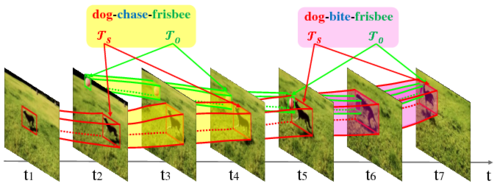### Task 3: Visual Relation Detection

Visual relation detection (VRD) is a novel research problem that is beyond the recognition of single object entity. It requires the visual system to understand many perspectives of two entities, including appearance, action, intention, and interactions between them. Specifically, it aims to detect instances of visual relations of interest in a video, where a visual relation instance is represented by a relation triplet <subject,predicate,object> with the bounding-box trajectories of the subject and object during the relation happening (as shown in Figure 1).Figure 1: Examples of visual relation instances in video. Adopted from "Video visual relation detection" (ACM MM'17)

This challenge offers the first large scale video dataset for VRD and intends to pave the way for the research on relation understanding in videos. In the task, participants are encouraged to develop methods that can not only recognize a wide range of visual relations from 80 object categories and 50 predicate categories, but also spatio-temporally localize various visual relation instances in a user-generated video. Note that the detection of social relations (e.g. “is parent of”) and emotional relations (e.g. “like”) is outside the scope of this task.

### Dataset

The dataset for this task consists of 10K user-generated videos from Flickr and annotations on 80 categories of object (e.g. "adult", "child", "dog", "table"), 42 categories of action predicate (e.g. "watch", "grab", and "hug") and 8 categories of spatial predicate (e.g. "in front of", "inside", "towards"). In the annotations, each relation instance is labeled and localized in the same way as the instances shown in Figure 1 (e.g. "dog-chase-frisbee" from t2 to t4). The training/validation/testing splits are 7,000, 835 and 2,165 videos. The videos and annotations can be downloaded directly from here.

### Evaluation Metric

We adopt Average Precision (AP) to evaluate the detection performance per video and finally calculate the mean AP (mAP) over all testing videos as the ranking score. In particular, we calculate AP similarly to that in the Pascal VOC challenge. To match a predicted relation instance $(\langle s,p,o\rangle^p,(\mathcal{T}_s^p,\mathcal{T}_o^p))$ to a ground truth $(\langle s,p,o\rangle^g,(\mathcal{T}_s^g,\mathcal{T}_o^g))$, we require:

• their relation triplets to be exactly same, i.e. $\langle s,p,o\rangle^p = \langle s,p,o\rangle^g$;
• their bounding-box trajectories overlap s.t. $\text{vIoU}(\mathcal{T}_s^p, \mathcal{T}_s^g) \geq 0.5$ and $\text{vIoU}(\mathcal{T}_o^p, \mathcal{T}_o^g) \geq 0.5$;
• the minimum overlap of the subject trajectory pair and the object trajectory pair $\text{ov}_{pg}=\min{(\text{vIoU}(\mathcal{T}_s^p, \mathcal{T}_s^g), \text{vIoU}(\mathcal{T}_o^p, \mathcal{T}_o^g))}$ is the maximum among those paired with the other unmatched ground truths $\mathcal{G}$, i.e. $\text{ov}_{pg} \geq \text{ov}_{pg'} (g' \in \mathcal{G})$.
The term $\text{vIoU}$ refers to the voluminal Intersection over Union.

The evaluation code used by the evaluation server can be found here. The number of predictions per video is limited up to 2000.

### Submission Format

Please use the following JSON format when submitting your results for the challenge:

The example above is illustrative. Comments must be removed in your submission. A sample submission file is available here.